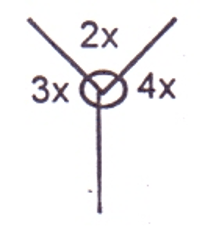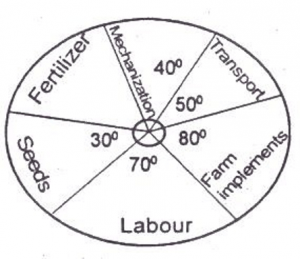## Mathematics 2017 Past Questions | JAMB

Study the following Mathematics past questions and answers for JAMBWAEC  NECO and Post-JAMB. Get prepared with official past questions and answers for upcoming examinations.

21. Simplify 4$$\sqrt{27}$$ + 5$$\sqrt{12}$$ − 3$$\sqrt{75}$$

• A. 7
• B. − 7
• C. − 7$$\sqrt{3}$$
• D. 7$$\sqrt{3}$$

22. A man covered a distance of 50 miles on his first trip, on a later trip he traveled 300 miles while going 3 times as fast. His new time compared with the old distance was?

• A. three times as much
• B. the same
• C. twice as much
• D. half as much

23.In the figure, find x
• A. 40o
• B. 55o
• C. 50o
• D. 60o

24. Divide 4x³ – 3x + 1 by 2x – 1

• A. 2x2 -x + 1
• B. 2x2 – x -1
• C. 2x2 + x + 1
• D. 2x2 + x -1

25. A car dealer bought a second-hand car for of 250,000 and spent N 70,000 refurbishing it. He then sold the car for N400,000. What is the percentage gain?

• A. 60%
• B. 32%
• C. 25%
• D. 20%

26. Find the number of ways that the letters of the word EXCELLENCE be arranged

• A. $$\frac{10!}{2!2!2!}$$
• B. $$\frac{10!}{4!2!}$$
• C. $$\frac{10!}{4!2!2!}$$
• D. $$\frac{10!}{2!2!}$$

27. If a rod 10cm in length was measured as 10.5cm, calculate the percentage error

• A. 5%
• B. 10%
• C. 8%
• D. 7%

28.  Evaluate $$\frac{0.00000231}{0.007}$$ and leave the answer in standard form

• A. 3.3 x 10-4
• B. 3.3 x 10-3
• C. 3.3 x 10-5
• D. 3.3 x 10-8

29. Find the principal which amounts to ₦ 5,500 at a simple interest in 5 years at 2% per annum

• A. ₦ 4,900
• B. ₦ 5,000
• C. ₦ 4,700
• D. ₦ 4,000

30.The pie chart shows the allocation of money to each sector in a farm. The total amount allocated to the farm is ₦ 80 000. Find the amount allocated to fertilizer

• A. ₦ 35, 000
• B. ₦ 40,000
• C. ₦ 25,000
• D. ₦ 20,000
Scroll to Top